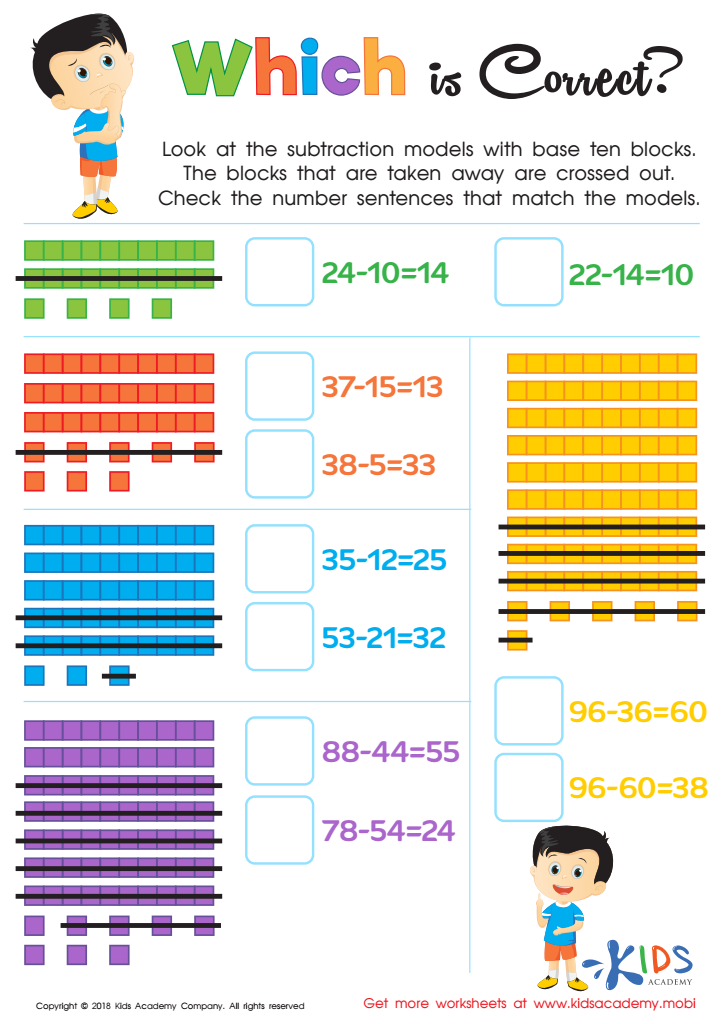# Easy Online Place Value Worksheets for Grade 1

11 filtered results

Clear all filters
Favorites
Online only

11 filtered results

Difficulty Level

Standards
Favorites
Online only
• Online only
• Place Value
• Easy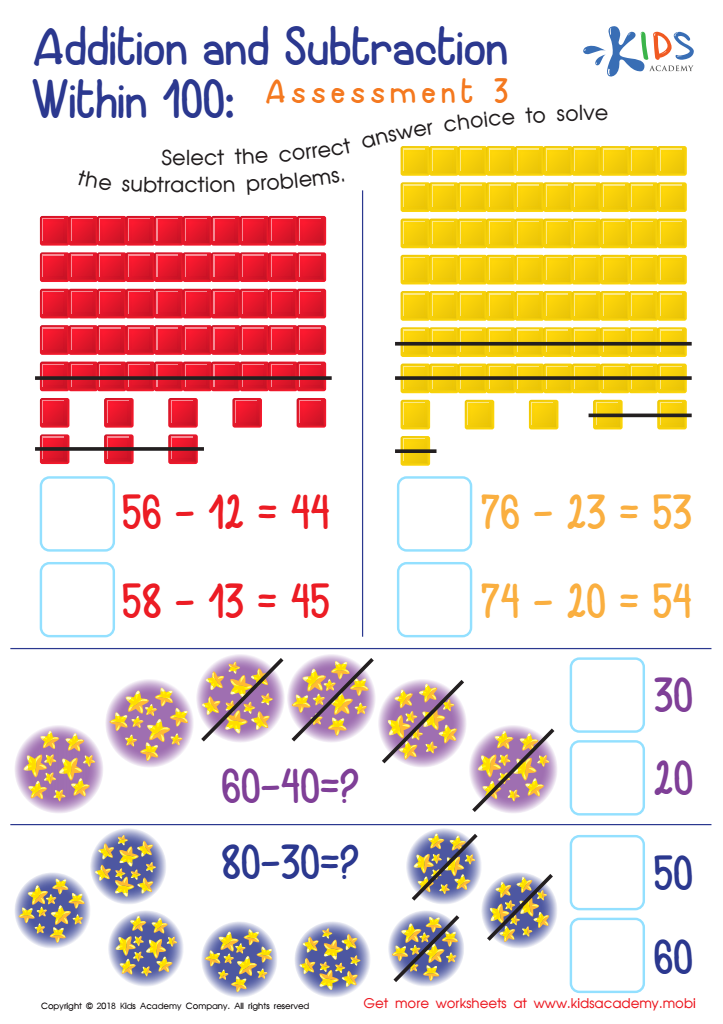Addition and Subtraction Within 1: Assessment 3 Worksheet
Help your kids count the base ten blocks in the picture. Then, count how many have been marked off. Ask them to select the correct answer choice to solve the subtraction problems based on the blocks counted. 80 words
Addition and Subtraction Within 1: Assessment 3 Worksheet
Worksheet
Addition and Subtraction Within 1: Assessment 3 Worksheet
Counting base ten blocks and subtraction skills are the two things your kids will have …
Place Value
Worksheet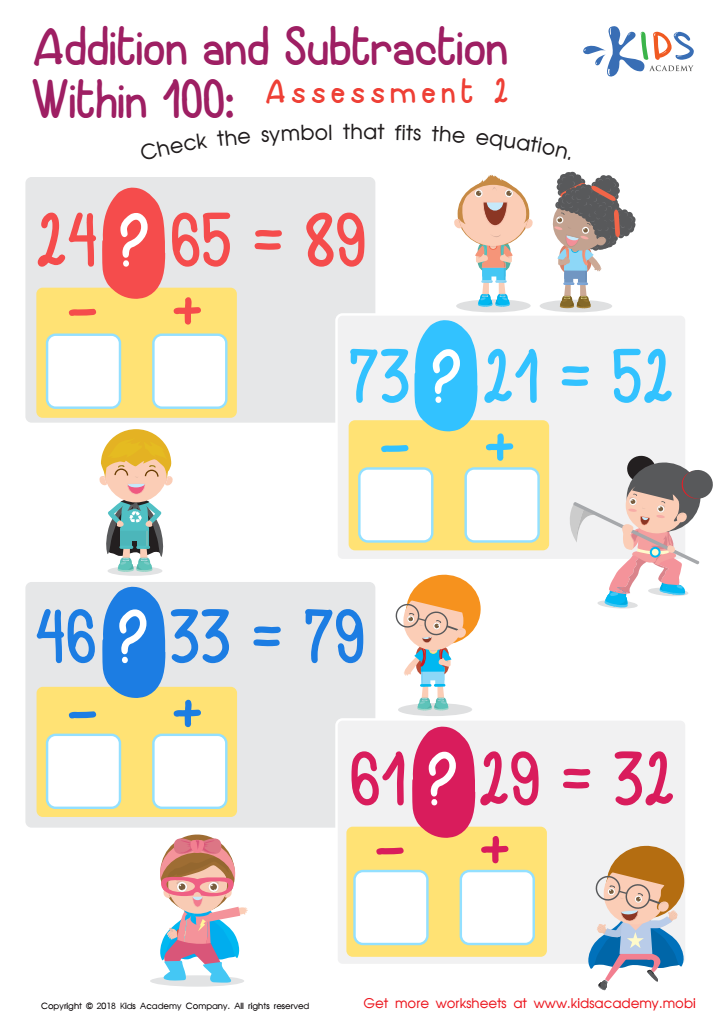Addition and Subtraction Within 1: Assessment 2 Worksheet
This worksheet is a great tool to help your students learn addition and subtraction. It contains four equations in a printable pdf. Ask your students to review the equation and determine if the symbol between them is a '+' or '-'. Guide them in selecting the correct symbols for each equation.
Addition and Subtraction Within 1: Assessment 2 Worksheet
Worksheet
Addition and Subtraction Within 1: Assessment 2 Worksheet
If you are teaching your students addition and subtraction in class now, this worksheet will …
Place Value
Worksheet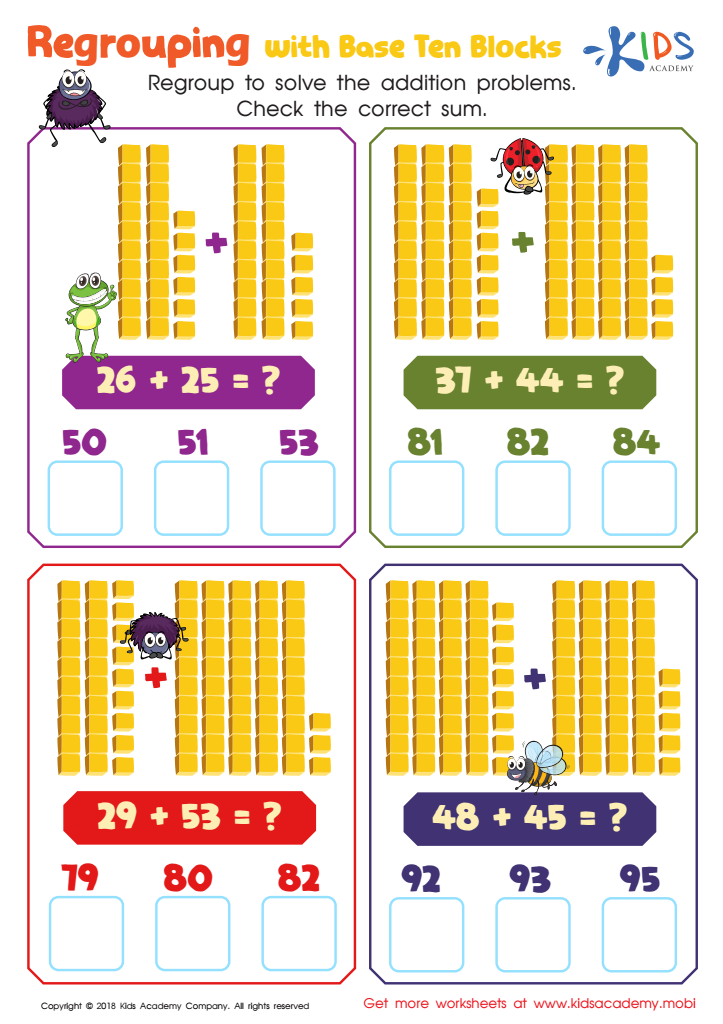Regrouping With Base Ten Blocks Worksheet
Challenge your child to regroup the base ten blocks and solve the four equations in this worksheet. This exercise requires counting and adding up in base ten. When they are done, help them check the correct sum.
Regrouping With Base Ten Blocks Worksheet
Worksheet
Regrouping With Base Ten Blocks Worksheet
The numbers in this simple worksheet need to be regrouped and solved. Your kids will …
Place Value
Worksheet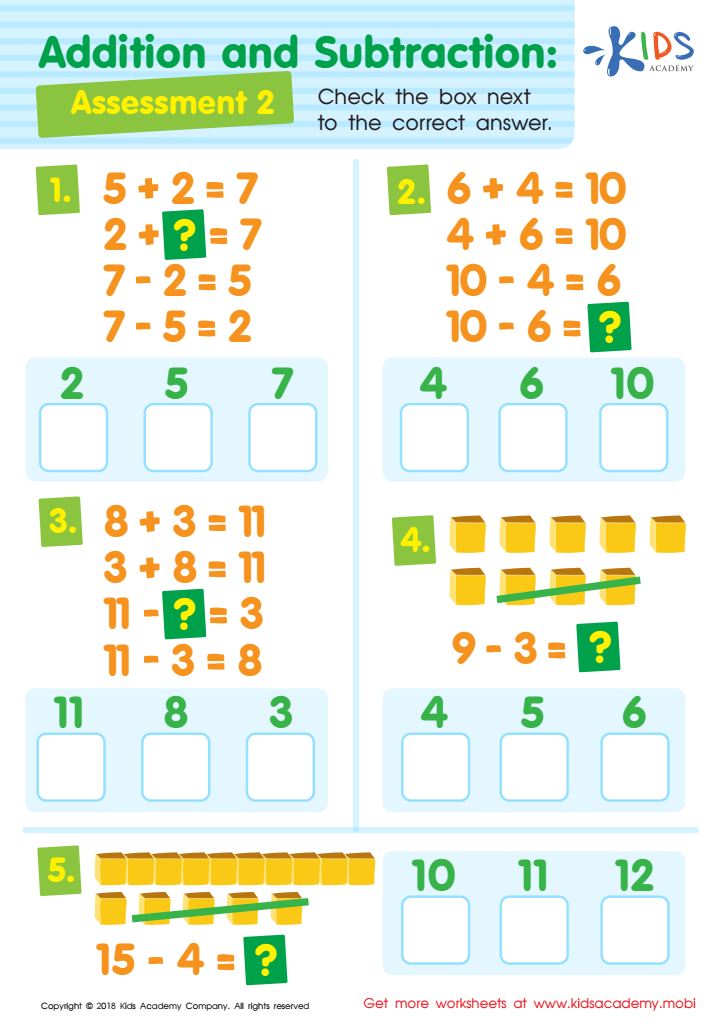Addition and Subtraction Assessment 2 Worksheet
As your child grows, math problems become harder. To keep their brains active and provide extra help if needed, use this math worksheet. It'll help them become more familiar with equations. Go through each one carefully with your child and have them check the box next to the right answer.
Addition and Subtraction Assessment 2 Worksheet
Worksheet
Addition and Subtraction Assessment 2 Worksheet
As your child progresses in age and class, the mathematical problems they have to solve …